BFOIT - Introduction to Computer Programming

# Practice Answers: Expressions and Plumbing Diagrams

1. Draw a plumbing diagram for a tip calculator which takes two numbers as inputs: the bill's total cost and the percentage of the tip.  The output of the expression (the amount of the tip) should be input to a println command box.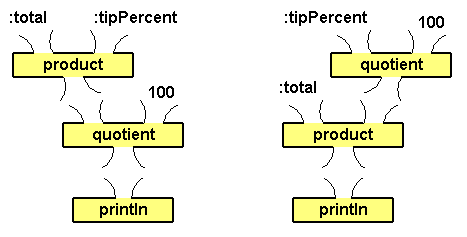Then write the procedure tipCalc which prints the tip amount...
 ``````to tipCalc :total :tipPercent println quotient (product :total :tipPercent) 100 end ``````
Here are some sample computations and some inputs without the tip percent left for you to check your program with.
 Bill Total Tip % Tip \$ \$10 15 1.50 \$15 20 3.00 \$32 16 5.12 \$22 18 3.96 \$47 17 7.99
2. The average of a bunch of numbers is the sum of the numbers divided by the number of numbers.

Draw a plumbing diagram for the expression which averages four numbers.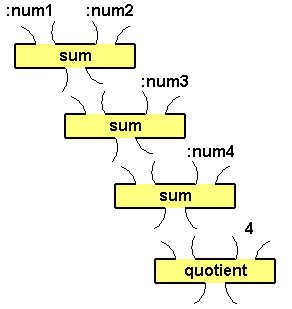And like the similar plumbing diagram solutions above, you can switch around the inputs to the sum boxes.

Write procedures to print the average of the numbers:
```
(a)  66, 10, 47  (Average: 41)
(b)  22, 87, 15, 41  (Average: 42)
(c)  83, 31, 72, 19, 6  (Average: 43) ```
 ``````to avg3num :num1 :num2 :num3 println quotient (sum (sum :num1 :num2) :num3) 3 end to avg4num :num1 :num2 :num3 :num4 println quotient (sum (sum (sum :num1 :num2) :num3) :num4) 4 end to avg5num :num1 :num2 :num3 :num4 :num5 println quotient (sum (sum (sum (sum :num1 :num2) :num3) :num4) :num5) 5 end ``````
3. Given Pythagoras' Theorem (the length of the hypotenuse of a right triangle is equal to the square root of the sum of the squares of each of its legs' lengths),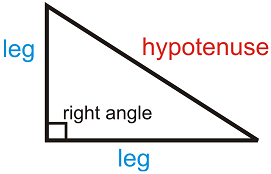Draw a plumbing diagram for this expression.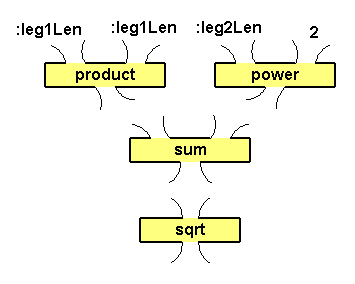Other correct plumbing diagrams may have two product boxes at the top, or two power boxes at the top, or the product and power boxes may be swapped with appropriate input changes.

Then write a procedure which prints the length of the hypotenuse of a right triangle, given the lengths of its legs.
 ``````to hypotenuseLength :leg1Len :leg2Len println sqrt sum (product :leg1Len :leg1Len) (power :leg2Len 2) end ``````
4. Write procedures which display the surface area and the volume of a sphere, given its radius.  Here are the formulas you need.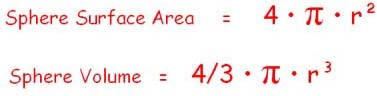`````` to sphereSurfaceArea :radius println product 4 (product 3.14159 (product :radius :radius)) end or to sphereSurfaceArea :radius println product 4 (product 3.14159 (power :radius 2)) end and to sphereVolume :radius println product (quotient 4 3) (product 3.14159 (product :radius (product :radius :radius)))   end or to sphereVolume :radius println product (quotient 4 3) (product 3.14159 (power :radius 3)) end ````````` Fill in the empty cells with the values your procedures print rounded to thousandths. Unit  Amount      Surface Area   (Unit2)    Volume   (Unit3) 1 12.566  4.189 2 50.265 33.510 3 113.097 113.097 4 201.062 268.083 ```
Note: There are calculators on the net that compute the surface area and volume of a sphere.  Here is one I played with.  One thing to be aware of is rounding.  In order for me to get the Logo procedure output to match the calculator I had to use 3.14159265359 for pi (instead of just 3.14159).